SSC  >  SSC CGL Tier-I Mock Test - 43

# SSC CGL Tier-I Mock Test - 43

Test Description

## 75 Questions MCQ Test SSC CGL Tier 1 Mock Test Series | SSC CGL Tier-I Mock Test - 43

SSC CGL Tier-I Mock Test - 43 for SSC 2022 is part of SSC CGL Tier 1 Mock Test Series preparation. The SSC CGL Tier-I Mock Test - 43 questions and answers have been prepared according to the SSC exam syllabus.The SSC CGL Tier-I Mock Test - 43 MCQs are made for SSC 2022 Exam. Find important definitions, questions, notes, meanings, examples, exercises, MCQs and online tests for SSC CGL Tier-I Mock Test - 43 below.
Solutions of SSC CGL Tier-I Mock Test - 43 questions in English are available as part of our SSC CGL Tier 1 Mock Test Series for SSC & SSC CGL Tier-I Mock Test - 43 solutions in Hindi for SSC CGL Tier 1 Mock Test Series course. Download more important topics, notes, lectures and mock test series for SSC Exam by signing up for free. Attempt SSC CGL Tier-I Mock Test - 43 | 75 questions in 60 minutes | Mock test for SSC preparation | Free important questions MCQ to study SSC CGL Tier 1 Mock Test Series for SSC Exam | Download free PDF with solutions
 1 Crore+ students have signed up on EduRev. Have you?
SSC CGL Tier-I Mock Test - 43 - Question 1

### The grid given below contains the codes of high performing employees from different IT companies. If the number starting with 8206 represents the code of the employees of Infosys, then how many employees of Infosys are high performers?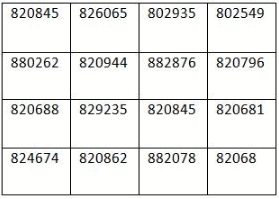SSC CGL Tier-I Mock Test - 43 - Question 2

### 7        5        4       3       2         1             6          8           9 \$         >       +       -       #         %            @        =            ×  What is the number indicated by the following symbols? + % X \$ =

SSC CGL Tier-I Mock Test - 43 - Question 3

### From the given alternatives select the word which cannot be formed using the letters of the given word COMPREHENSION

Detailed Solution for SSC CGL Tier-I Mock Test - 43 - Question 3

There is only one ‘i’ in the word.

SSC CGL Tier-I Mock Test - 43 - Question 4

Find the missing term: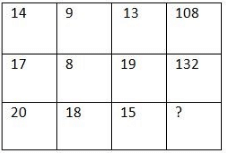Detailed Solution for SSC CGL Tier-I Mock Test - 43 - Question 4

14 + 9 + 13 = 36 × 3 = 108
17 + 8 + 19 = 44 × 3 = 132
20 + 18 + 15 = 53 × 3 = 159

SSC CGL Tier-I Mock Test - 43 - Question 5

From among the given alternatives select the one in which the set of numbers is most like the set of numbers given in the question.

Given set: (13, 20, 9)

Detailed Solution for SSC CGL Tier-I Mock Test - 43 - Question 5

13 + 20 + 9 = 42
Similarly,
17 + 14 + 11= 42

SSC CGL Tier-I Mock Test - 43 - Question 6

Find the missing term.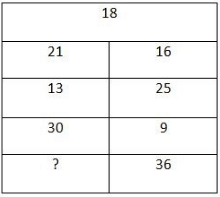Detailed Solution for SSC CGL Tier-I Mock Test - 43 - Question 6

The pattern is,
18 + 3 = 21, 18 – 2 = 16
21 + 4 = 25, 16 – 3 = 13
25 + 5 = 30, 13 – 4 = 9
30 + 6 = 36, 9 – 5 = 4

SSC CGL Tier-I Mock Test - 43 - Question 7

Directions

Study the following question and based on this, answer the following questions.

C is to the west of I. D is 5m to the north of C. E is 5m to the north of D. F is 9m to the west of E. G is 5m to the south of F. H is 12m to the East of D.

Q. How far is G from H?

Detailed Solution for SSC CGL Tier-I Mock Test - 43 - Question 7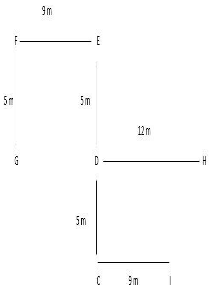GH = GD + DH
= 9 + 12 = 21m

SSC CGL Tier-I Mock Test - 43 - Question 8

Directions

Study the following question and based on this, answer the following questions.

C is to the west of I. D is 5m to the north of C. E is 5m to the north of D. F is 9m to the west of E. G is 5m to the south of F. H is 12m to the East of D.

Q. H is in which direction with respect to E?

Detailed Solution for SSC CGL Tier-I Mock Test - 43 - Question 8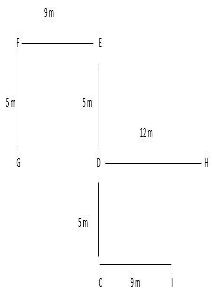SSC CGL Tier-I Mock Test - 43 - Question 9

E is the father of his only child D and Q is grandson of F, who is wife of E. H is mother of T and only daughter-in-law of E. How is D related to T?

Detailed Solution for SSC CGL Tier-I Mock Test - 43 - Question 9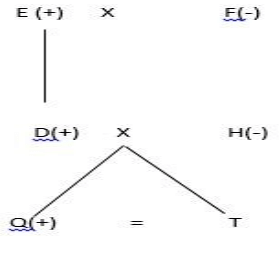SSC CGL Tier-I Mock Test - 43 - Question 10

Directions

In each of the questions below are given two/three statements followed by two conclusions numbered I, and II. You have to take the given statements to be true even if they seem to be at variance with commonly known facts. Read all the conclusions and then decide which of given conclusions logically follows from the given statements disregarding commonly known facts.

Statement: No cooler is a fan.

All fans are ACs.

No AC is a button.

Conclusion: No fan is a button.

Some ACs are cooler.

Detailed Solution for SSC CGL Tier-I Mock Test - 43 - Question 10

All fans are ACs(A) +  No AC is a button(E) ⇒ No fan is a button(E). Hence conclusion I follows.
No cooler is a fan(E) + All fans are ACs(A) ⇒ Some ACs are not coolers(O). Hence conclusion II will not follow.

SSC CGL Tier-I Mock Test - 43 - Question 11

Directions

In each of the questions below are given two/three statements followed by two conclusions numbered I, and II. You have to take the given statements to be true even if they seem to be at variance with commonly known facts. Read all the conclusions and then decide which of given conclusions logically follows from the given statements disregarding commonly known facts.

Statement: Some letters are papers

All papers are objects.

Some objects are subjects.

Conclusion: Some papers are not subjects.

All subjects are objects

Detailed Solution for SSC CGL Tier-I Mock Test - 43 - Question 11

All papers are objects(A) + Some objects are subjects(I) ⇒ No conclusion. Hence conclusion I will not follow.
Some objects are subjects(I) ⇒ conversion ⇒ Some subjects are objects(I). Hence conclusion II will not follow

SSC CGL Tier-I Mock Test - 43 - Question 12

If the English alphabetical series in written from right to left, which Letter will be the 6th letter to the right of the 9th letter from the left of the last but one letter from the right.

SSC CGL Tier-I Mock Test - 43 - Question 13

Pick the odd one out:

Detailed Solution for SSC CGL Tier-I Mock Test - 43 - Question 13

Except MS office, all are operating systems

SSC CGL Tier-I Mock Test - 43 - Question 14

Which one the following comes in the place of (?)

17           49           ?              592         1578

Detailed Solution for SSC CGL Tier-I Mock Test - 43 - Question 14

17 × 3 – 2 = 49
49 × 4 + 2 = 198
198 × 3 – 2 = 592
394 × 4 + 2 = 1578

SSC CGL Tier-I Mock Test - 43 - Question 15

How many such pairs of letters are there in the word ‘APTITUDE’, each of which has many letters between them in the word as they have between them in the English alphabetical series?

Detailed Solution for SSC CGL Tier-I Mock Test - 43 - Question 15

TU, DE, EI

SSC CGL Tier-I Mock Test - 43 - Question 16

Eight friends S, T, U, V, W, X, Y and Z are sitting in a straight line facing north, but not necessarily in the same order. U sits third from the left end. Only one person sits between U and Y. W sits on immediate left of T. Z sits on the immediate right of X. Z sits on the left of V. O only one person sits between V and S. V is not on the left S. Who among the following sits between U and Y?

Detailed Solution for SSC CGL Tier-I Mock Test - 43 - Question 16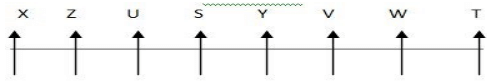SSC CGL Tier-I Mock Test - 43 - Question 17

In a certain coded language DRUM is coded as AOVI and VARY is coded as UBOU, then what is the code of AUTO?

Detailed Solution for SSC CGL Tier-I Mock Test - 43 - Question 17

DRUM –AOVI
VARY – UBOU
Then, AUTO – BVOP
Every vowel is coded as next consonant and every consonants is coded as previous vowel.

SSC CGL Tier-I Mock Test - 43 - Question 18

Arrange the following words in a meaningful order.

A. Spawn          B. Frog               C. Froglet                D. Tadpole

Detailed Solution for SSC CGL Tier-I Mock Test - 43 - Question 18

Spawn  →      Tadpole  →      Froglet  →  Frog

SSC CGL Tier-I Mock Test - 43 - Question 19

72 : 180 : : 60 : ?

Detailed Solution for SSC CGL Tier-I Mock Test - 43 - Question 19

72 × 2.5 = 180
Similarly 60 × 2.5 = 150

SSC CGL Tier-I Mock Test - 43 - Question 20

Interchanging which two operational symbols will make the given equation true?

36 × 5 - 84 ÷ 4 + 50 = 151

Detailed Solution for SSC CGL Tier-I Mock Test - 43 - Question 20

If we interchange (+ and -) then equation will become correct → 36 × 5 + 84 ÷ 4 - 50
= 180 + 21 - 50 → 201 - 50 = 151

SSC CGL Tier-I Mock Test - 43 - Question 21

Find the number of triangles in the given figure.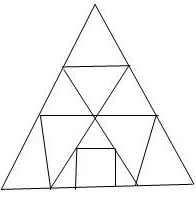Detailed Solution for SSC CGL Tier-I Mock Test - 43 - Question 21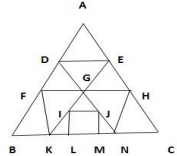The triangles are: ADE, GDE, DFG, EGH, FKB, FGK, GIJ, GHN, HNC, ILK, JMN, AFH, DBN, GKN, EKC, ABC

SSC CGL Tier-I Mock Test - 43 - Question 22

The columns and rows of Matrix I are numbered from 0 to 4 and that of Matrix II are numbered from 5 to 9. A letter from these matrices can be represented first by its row and next by its column. e.g. ‘A’ can be represented by 03, 12 etc. and ‘N’ can be represented by 56, 65 etc. Identify the set for the word DRAW.

MATRIX – I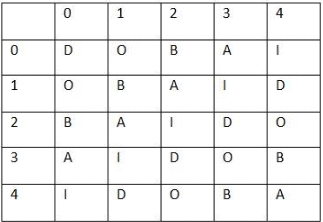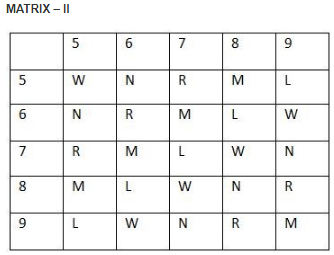Detailed Solution for SSC CGL Tier-I Mock Test - 43 - Question 22

D – 00, 14, 23, 32, 41
R – 57, 66, 75, 89, 98
A – 03, 12, 21, 30, 44
W – 55, 69, 78, 87, 96

SSC CGL Tier-I Mock Test - 43 - Question 23

In the question one part of the problem figure is subtracted. Select the portion that shows the correct shape by the subtraction.

Question figure: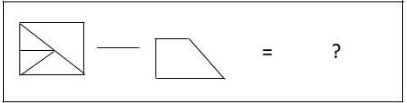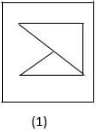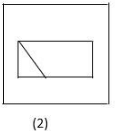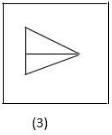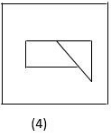SSC CGL Tier-I Mock Test - 43 - Question 24

In the following questions select the related figure from the given alternatives.

Question figure: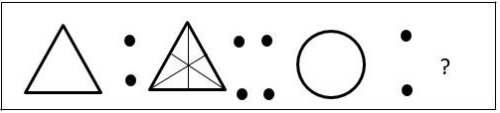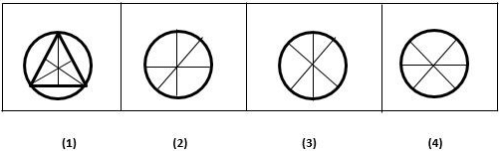Detailed Solution for SSC CGL Tier-I Mock Test - 43 - Question 24

From the first unit to second unit three line segments are added in a set pattern.

SSC CGL Tier-I Mock Test - 43 - Question 25

Four positions of dice are given below. Which letter will be opposite to D?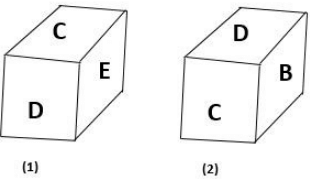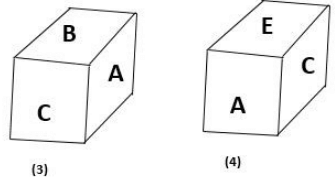Detailed Solution for SSC CGL Tier-I Mock Test - 43 - Question 25

The letters A, B, C, D and E are on the adjacent faces of face C. therefore, some other letter lies opposite ‘C’. The letters B, C and E are on the adjacent faces of face D. therefore, A lies opposite D.

SSC CGL Tier-I Mock Test - 43 - Question 26

India’s longest bridge connects

Detailed Solution for SSC CGL Tier-I Mock Test - 43 - Question 26

PM Narendra Modi on 26 May inaugurated India’s longest bridge, spanning 9.3 km across the Brahmaputra, promising to turn the north-east into a showpiece of infrastructure development. The road bridge, connecting Dhola in Arunchal Pradesh to Sadiya in Assam.

SSC CGL Tier-I Mock Test - 43 - Question 27

India’s rank in Global Innovation Index is

Detailed Solution for SSC CGL Tier-I Mock Test - 43 - Question 27

India move up six places to the 60 position in a survey of innovative capacity among 130 nations on the Global Innovation Index 2017.

SSC CGL Tier-I Mock Test - 43 - Question 28

In which city in India first three cases of Zika virus has been confirmed by the World Health Organisation (WHO)?

Detailed Solution for SSC CGL Tier-I Mock Test - 43 - Question 28

The World Health Organisation (WHO) has confirmed the first three cases of Zika virus in India, as having been reported from the city in Gujarat

SSC CGL Tier-I Mock Test - 43 - Question 29

Who has been awarded with Man Booker Prize recently?

Detailed Solution for SSC CGL Tier-I Mock Test - 43 - Question 29

Israeli author David Grossman has won the Man Booker International Prize for his novel A Horse Walks into a Bar. His novel is about a failing standup comic.

SSC CGL Tier-I Mock Test - 43 - Question 30

Every year first Sunday of May is observed as which of the following days?

Detailed Solution for SSC CGL Tier-I Mock Test - 43 - Question 30

World laughter Day takes place on the first Sunday of May of every year. The first celebration was on January 10, 1998, in Mumbai, India, and was arranged by Dr. Madan Kataria, founder of the worldwide Laughter Yoga movement.

SSC CGL Tier-I Mock Test - 43 - Question 31

Kanger Ghati National Park is situated in the state of

Detailed Solution for SSC CGL Tier-I Mock Test - 43 - Question 31

Kanger Ghati National Park was declared a national park in 1982 by the Government of India. Among the various protected areas in the country, the Kanger Valley National Park near Jagdalpur, in the Bastar region of Chhattisgarh is one of the most beautiful and densest national parks, well known for its biodiversity with picturesque landscape, magnificent waterfalls, very famous subterranean geomorphologic limestone caves, and home for the Bastar hill myna, the state bird of Chhattisgarh.

SSC CGL Tier-I Mock Test - 43 - Question 32

Which is the second highest military award for gallantry during wartime?

Detailed Solution for SSC CGL Tier-I Mock Test - 43 - Question 32

The Maha Vir chakra is the second highest military decoration in India, after the Param Vir Chakra, and is awarded for acts of conspicuous gallantry in the presence of the enemy, whether on land, at sea or in the air.

SSC CGL Tier-I Mock Test - 43 - Question 33

‘Rand’ is the currency of which of the following countries?

Detailed Solution for SSC CGL Tier-I Mock Test - 43 - Question 33

Nigeria – Naira
South Africa – Rand
Namibia – Shilling
Ethiopia – Birr

SSC CGL Tier-I Mock Test - 43 - Question 34

Increase in the focal length of eye lens and Stiffening of ciliary muscles is caused by

Detailed Solution for SSC CGL Tier-I Mock Test - 43 - Question 34

A person suffering from hypermetropia can see the distant objects clearly but not the near object.

SSC CGL Tier-I Mock Test - 43 - Question 35

Which of the following disease is caused by the deficiency of iodine in food?

Detailed Solution for SSC CGL Tier-I Mock Test - 43 - Question 35

Goitre is the disease caused by the deficiency of iodine in food. In this disease the shape of the thyroid gland enlarges abnormally.

SSC CGL Tier-I Mock Test - 43 - Question 36

The India’s First Woman Governor of India is

Detailed Solution for SSC CGL Tier-I Mock Test - 43 - Question 36

Sarojini Naidu was the first female to become the governor of an Indian state. She governed Uttar Pradesh from 15 August 1947 to 2 March 1949.

SSC CGL Tier-I Mock Test - 43 - Question 37

Mercalli scale is the instrument used for measuring the intensity of ___________

Detailed Solution for SSC CGL Tier-I Mock Test - 43 - Question 37

The Mercalli intensity scale is a seismic scale used for measuring the magnitude and intensity of an earthquake. It measures the effects of an earthquake.

SSC CGL Tier-I Mock Test - 43 - Question 38

The first Chairman of Finance commission was

Detailed Solution for SSC CGL Tier-I Mock Test - 43 - Question 38

The First Finance Commission of India was appointed in 1951, for the period 1952-57 by the President of India and was chaired by K.C.Neogy.

SSC CGL Tier-I Mock Test - 43 - Question 39

Nadir Shah took away Kohinoor Diamond during the period of  _____________

Detailed Solution for SSC CGL Tier-I Mock Test - 43 - Question 39

In 1738-39, Nadir Shah raided India and took away Thakht-i-Taus (The Peacock throne) and Kohinoor Diamond during the period of Muhammad Shah.

SSC CGL Tier-I Mock Test - 43 - Question 40

The Polymer used for Electrical insulation buttons is

Detailed Solution for SSC CGL Tier-I Mock Test - 43 - Question 40

Bakelite was used for its electrical non-conductivity and heat-resistant properties in electrical insulators, radio and telephone casings and such diverse products as kitchenware, jewellery, pipe stems, children toys and firearms.

SSC CGL Tier-I Mock Test - 43 - Question 41

The Emblem of the Chola Dynasty was

Detailed Solution for SSC CGL Tier-I Mock Test - 43 - Question 41

The Tiger or Jumping Tiger was the royal emblem of the Cholas and was depicted on coins, seals and banners.

SSC CGL Tier-I Mock Test - 43 - Question 42

Match the following Important Books on Economics with their respective Authors.

Books on Economics                                  Author

(i) The Wealth of Nations                           A. Irwin Fisher

(ii) Money Illusion                                      B. Adam Smith

(iii) The Value and Capital                         C. J.M.Keynes

(iv) General Theory of Employment          D. Hicks

SSC CGL Tier-I Mock Test - 43 - Question 43

Which of the following metal has the highest Melting point?

Detailed Solution for SSC CGL Tier-I Mock Test - 43 - Question 43

Of all the metals in Pure form, tungsten has the highest melting point, Lowest vapour pressure and the highest tensile strength.

SSC CGL Tier-I Mock Test - 43 - Question 44

The Study of lizards is known as

Detailed Solution for SSC CGL Tier-I Mock Test - 43 - Question 44

Study of Lizards is Saurology
-Study of Pulse and arterial pressure is Sphygmology
-Study of serum, interaction of antigen and antibodies in the blood is Serology
-Study of parasites is Parasitology.

SSC CGL Tier-I Mock Test - 43 - Question 45

Which of the following rock is not sedimentary rock?

Detailed Solution for SSC CGL Tier-I Mock Test - 43 - Question 45

Granite is a common type of Igneous rock that is granular and phaneritic in nature.

SSC CGL Tier-I Mock Test - 43 - Question 46

Article __ of the Indian Constitution ensures Abolition of Untouchability.

Detailed Solution for SSC CGL Tier-I Mock Test - 43 - Question 46

Under Article 17, Untouchability is abolished and its practice in any form is forbidden. The enforcement of any disability arising out of Untouchability shall be an offence punishable in accordance with law.

SSC CGL Tier-I Mock Test - 43 - Question 47

Who was the first king to have the image of Lord Buddha inscribed on his coins?

Detailed Solution for SSC CGL Tier-I Mock Test - 43 - Question 47

Kanishka's coins portray images of Indian, Greek, Iranian and even Sumero – Elamite divinities, demonstrating the religious syncretism in his beliefs. Kanishka's coins from the beginning of his reign bear legends in Greek language and script and depict Greek divinities

SSC CGL Tier-I Mock Test - 43 - Question 48

DNA fingerprinting is a technique used for the detection of:

Detailed Solution for SSC CGL Tier-I Mock Test - 43 - Question 48

The first methods for finding out genetics used for DNA profiling involved RFLP analysis. DNA is collected from cells, such as a blood sample, and cut into small pieces using a restriction enzyme (a restriction digest).

SSC CGL Tier-I Mock Test - 43 - Question 49

Foramen Magnum is an aperture of

Detailed Solution for SSC CGL Tier-I Mock Test - 43 - Question 49

The foramen magnum (Latin: great hole) is a large oval opening (foramen) in the occipital bone of the skull in humans and various other animals. It is one of the several oval or circular openings (foramina) in the base of the skull.The foramen magnum is a very important feature in bipedal mammals.

SSC CGL Tier-I Mock Test - 43 - Question 50

Fermentation is a

Detailed Solution for SSC CGL Tier-I Mock Test - 43 - Question 50

Fermentation is a metabolic process that converts sugar to acids and gases, or alcohol. It occurs in yeast and bacteria, and also in oxygen-starved muscle cells, as in the case of lactic acid fermentation. Fermentation is also used more broadly to refer to the bulk growth of microorganisms on a growth medium, often with the goal of producing a specific chemical product.

SSC CGL Tier-I Mock Test - 43 - Question 51

At present, Naveen is six years younger than Mohit. Mohit’s age fifteen years hence will be equal to thrice that of Naveen three years ago. What will be the sum of their present age?

Detailed Solution for SSC CGL Tier-I Mock Test - 43 - Question 51

Mohit = x
Naveen = (x-6)
15 years hence, Mohit’s age = (x + 15) years
3 years ago, Naveen = x - 6 - 3
= (x - 9) years
Now,  (x+15) = 3 (x-9)
x + 15 = 3x - 27
42 = 2x
x = 21 years
Hence, Mohit = 21 years
Naveen = 21 - 6 = 15 years
Sum of the present ages of Mohit and Naveen = 21 + 15 = 36 years

SSC CGL Tier-I Mock Test - 43 - Question 52

The distance between two places P and Q is 550 km. A car departs from place P for place Q at a speed of 60 km/hr. at 5 am. Another car departs from place Q for place A at a speed of 40 km/hr at 10 am. At what time will both the car meet each other?

Detailed Solution for SSC CGL Tier-I Mock Test - 43 - Question 52

Distance travelled by the first car in 5 hours = 60 × 5 = 300 km
Remaining distance = 550 - 300
= 250 km
Time for both cars to meet = distance/(relative speed )
= 250/(60+40) = 250/100
= 21/2 hrs = 2 hrs 30 min
∴ Both the cars will meet after 2 hrs 30 min means (10 am + 2 hrs 30 min) = 12 hrs 30 min

SSC CGL Tier-I Mock Test - 43 - Question 53

Amit started a business by investing Rs. 60,000. After 4 months, Harish joined him with a capital of Rs. 48,000. At the end of the years Harish received Rs. 2800 as profit. What is the total profit at the end of the year?

Detailed Solution for SSC CGL Tier-I Mock Test - 43 - Question 53

Ratio of Amit’s profit to Harish’s profit = 60 × 12 : 48 × 8
= 15 : 8
Now, lets Amit’s profit be 15x and that of Harish be 8x.
Given, 8x = 2800
x = 350
∴ Total profit = 15x + 8x
= 23x = 23 × 350
= 8050

SSC CGL Tier-I Mock Test - 43 - Question 54

Abhishek, Rohit and Pankaj working together completed a job in 20 days. The ratio of their efficiencies is 3 : 4 : 5. How many days will be required by the fastest worker alone to complete the whole work?

Detailed Solution for SSC CGL Tier-I Mock Test - 43 - Question 54

Ratio of efficiencies = 3 : 4 : 5
Ratio of time required = 1/3 : 1/4 : 1/5
= 20 : 15 : 12
Suppose Abhishek, Rohit and Pankaj complete the work in 20x, 15x, 12x days respectively.
Then,
1/20x + 1/15x + 1/12x = 1/20
(3x+4x+5x)/60x
12/60x = 1/20
x = (12×20)/60 = 4 days
Hence, the fastest worker (Pankaj) can complete the work = 12x
= 12 × 4
= 48 days

SSC CGL Tier-I Mock Test - 43 - Question 55

A man rows to a place 221 km away and comes back to the starting point. If the speed of the stream is 2 km/hr and the speed of the boat in still water is 15 km/hr. Then what is the total time taken by him?

Detailed Solution for SSC CGL Tier-I Mock Test - 43 - Question 55

221/((15+2)) + 221/((15-2)) = 13 + 17 = 30 hrs

SSC CGL Tier-I Mock Test - 43 - Question 56

A shopkeeper marks the price of an article Rs. 3200. What will be the selling price if he allows two successive discounts of 30% each?

Detailed Solution for SSC CGL Tier-I Mock Test - 43 - Question 56

Marked price
∴ SP = 3200 × 70/100 × 70/100 = Rs. 1568

SSC CGL Tier-I Mock Test - 43 - Question 57

Two Poles are 12 metres apart horizontally and the height of one is double that of the other. If from the mid-point of the line joining their feet, an observer finds the angular elevations of their tops to be complementary. Then the height of the shorter post is:

Detailed Solution for SSC CGL Tier-I Mock Test - 43 - Question 57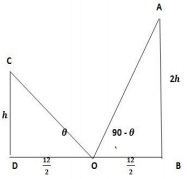Let AB = taller pole
CD = shorter pole = h
OB = OD =( 12)/2 = 6m
In ∆OCD,
tanθ = h/6 ............................(i)
In ∆AOB
tan 〖(90- θ)〗   = 2h/6 = h/3
cotθ=  h/3   .............................(ii)
Multiplying (i) and (ii), we get
tanθ ×cotθ = h/6  ×  4/3
1 = h²/18
h² = 18
h=3√2 m

SSC CGL Tier-I Mock Test - 43 - Question 58

In a mixture of 85 litres, the ratio of milk to water is 2 : 3. If this ratio is to be 1 : 3, then what is the quantity of water needed to be added?

Detailed Solution for SSC CGL Tier-I Mock Test - 43 - Question 58

Quantity of milk in the mixture = 85 × 2/5 = 34 liters
Quantity of water in the mixture = 85 × 3/5 = 51 liters
Let x liters water be added to the mixture then,
34/(51+ x) = 1/3
102 = 51 + x
x = 102 - 51
= 51 litres

SSC CGL Tier-I Mock Test - 43 - Question 59

The ratio of P’s to Q’s salary is 5 : 7. If Q’s salary is increased by 35%, his total salary becomes Rs. 52,920. What is the salary of P?

Detailed Solution for SSC CGL Tier-I Mock Test - 43 - Question 59

Let the salary of P and Q be 5x and 7x respectively.
Then,
7x × 135/100 = 52,920
x = (52920×100)/(135×7)
= Rs. 5600
∴ P’s salary = 5x
= 5 × 5600
= Rs. 28000

SSC CGL Tier-I Mock Test - 43 - Question 60

How much water must be added to a cask which contain 32 litre of milk at a cost price of Rs 45 per litre so that the cost of milk reduces to Rs. 40 per litre?

Detailed Solution for SSC CGL Tier-I Mock Test - 43 - Question 60

Let x litre water be added in the mixture.
Then,
(32+ x) 40 = 45 × 32
1280 + 40x = 1440
40x = 160
x = 4
Therefore, 4 litre water must be added in the mixture.

SSC CGL Tier-I Mock Test - 43 - Question 61

A train running at the speed of 35 metre per second crosses a bridge in 30 seconds. Another which is 130 metre shorter than the previous train crosses the same bridge at the speed of 40 metre per second. Find the time taken by the second train to cross the bridge?

Detailed Solution for SSC CGL Tier-I Mock Test - 43 - Question 61

Let the length of the bridge be x m and that of the first train be y m
(x+y)/35 = 30
x+y = 1050
Again = (x+(y-130))/40 = t
(x+y-130)/40
(1050-130)/40 = 920/40
= 23 seconds

SSC CGL Tier-I Mock Test - 43 - Question 62

The Value of (sin38˚)/(cos52˚)+  (cos12˚)/(sin78˚)- 4cos²60˚ is

Detailed Solution for SSC CGL Tier-I Mock Test - 43 - Question 62

(sin38˚)/(cos52˚)+  (cos12˚)/(sin78˚)- 4cos²60˚
(sin (90-52)˚)/(cos52˚)+  (cos (90-78)˚)/(sin78˚)- 4cos²60˚
(cos52˚)/(cos52˚)+  (sin78˚)/(sin78˚)- 4cos²60˚
⇒1 + 1 - 4×(1/2)²
⇒1

SSC CGL Tier-I Mock Test - 43 - Question 63

A tradesman offers 8% discount on the marked price and 1 article free with every 19 article bought and still gains 35%. If the marked price is 35% more than the cost price of Rs. 100. Then what is the selling price?

Detailed Solution for SSC CGL Tier-I Mock Test - 43 - Question 63

Total discount = 8% + 1/((19+1)) × 100
= 8% + 1/20 × 100
= 8 + 5
= 13%
CP = Rs. 100
MP =  Rs. 135
SP = 135× 88/100 × 135/100
= Rs. 160.38

SSC CGL Tier-I Mock Test - 43 - Question 64

In physics examination a student scored 45% marks in the first paper out of 160. How much percent marks should he score in the second paper out of 140 marks if he is to get an overall of 66% marks?

Detailed Solution for SSC CGL Tier-I Mock Test - 43 - Question 64

Marks obtained in first paper = 45/100 × 160 = 72
Marks to be obtained in both the papers (160+140) × 66/100
= 198
∴ Marks to be obtained necessarily is second paper = 198 - 72
= 126
∴ Percentage of marks to be obtained in second paper = 126/140 × 100 = 90%

SSC CGL Tier-I Mock Test - 43 - Question 65

A rectangle with a diagonal of length 28m is inscribed in a circle. Find the area of a circle?

Detailed Solution for SSC CGL Tier-I Mock Test - 43 - Question 65

Radius of circle = 28/2 = 14 m
Area of circle =  π/4 r²
= 22/7 × 14 × 14
= 616 cm²

SSC CGL Tier-I Mock Test - 43 - Question 66

A wire is moulded into a circle of radius 21 cm. Then the wire is bent and shaped into a rectangle of length 36 m. Find the area of the rectangle thus formed?

Detailed Solution for SSC CGL Tier-I Mock Test - 43 - Question 66

Circumference of circle = Perimeter of a rectangle = 2πr
= 2 × 22/7 × 21
= 132 m
Perimeter of a rectangle = 2(l + b)
132 = 2(l + b)
36 + b = 66
B= 66 – 36 = 30 m
∴ Area of the rectangle =  l × b = 36 × 30
= 1080 m²

SSC CGL Tier-I Mock Test - 43 - Question 67

Suraj is one third as good as workman as Pankaj and together they finish a piece of work in 36 days. The number of days taken by Suraj alone to finish the work is

Detailed Solution for SSC CGL Tier-I Mock Test - 43 - Question 67

Panjkaj’s 1 day work = 1/x
Suraj’s 1 day’s work = 1/3x
Then,
1/x + 1/3x = 1/36
4/3x = 1/36
x = (4×36)/3 = 48 days
Suraj alone can complete the work in 3×48 = 144 days

SSC CGL Tier-I Mock Test - 43 - Question 68

Find the value of  X in the given figure: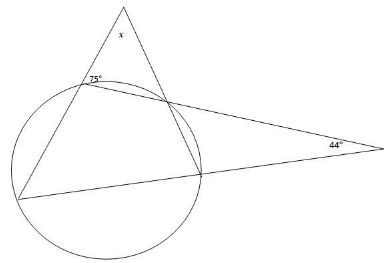Detailed Solution for SSC CGL Tier-I Mock Test - 43 - Question 68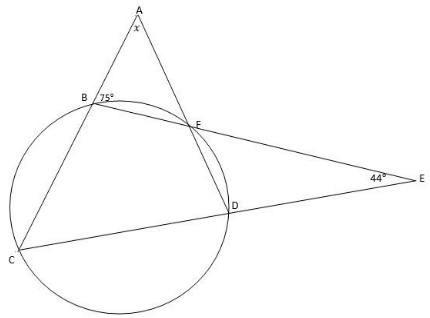In the given figure
∠ABF + ∠CBF = 180˚ (Angles in a straight line)
75˚ + ∠CBF = 180˚
∠CBF = 105˚
Now, in ∆CBE
∠CBE + ∠BCE + ∠CEB = 180˚
105˚ + ∠BCE + 44˚ = 180˚
∠BCE = 180˚ - (105˚+ 44˚)
∠BCE = 31˚
∠BCE + ∠DFB = 180˚ (Sum of opposite sides of Cyclic Quadrilateral)
∠DFB = 180˚ - 31˚ = 149˚
∠DFB + ∠AFB = 180˚
∠AFB = 180˚ - 149˚ = 31˚
In ∆ABF
∠AFB + ∠FAB + ∠AFB = 180˚
∠FAB = 180˚-(75˚+31˚) = 74˚

SSC CGL Tier-I Mock Test - 43 - Question 69

A man takes a total of 5 hrs 30 min by walking to a certain place and coming back by cycle. He would have gained 2 hrs 30 min by cycling both the ways. The time he would take to walk both ways is

Detailed Solution for SSC CGL Tier-I Mock Test - 43 - Question 69

By cycling + by walking = 5 hrs 30 min .......... (i)
One way by cycling = (5 hrs 30 min 〖- 2 hrs 30 min〗)/2
= 1 hrs 30 min
∴ By walking both ways = 2 (5 hrs 30 min - 1 hrs 30 min) = 8 hrs.

SSC CGL Tier-I Mock Test - 43 - Question 70

The slope of the equation 24x+8y=56 is

Detailed Solution for SSC CGL Tier-I Mock Test - 43 - Question 70

For the equation
y=mx+c
Where ‘m’ is the slope
24x+8y=56
8y= -24x+56
y=  (-24x)/8+  56/8
y= -3x+7  Slope = -3

SSC CGL Tier-I Mock Test - 43 - Question 71

Find the value of ‘x’ in the given expression

(49/16)× (64/343)x-1 = 4/7

Detailed Solution for SSC CGL Tier-I Mock Test - 43 - Question 71

(49/16)^x× (64/343)^(x-1) = 4/7
⇒(7/4)^2x× (4/7)^(3x-3) = 4/7

⇒(4/7)〗^(-2x)× (4/7)^(3x-3) = 4/7
⇒ -2x+3x-3=1 ⇒ x = 4

SSC CGL Tier-I Mock Test - 43 - Question 72

Directions

Study the graph and answer the following questions: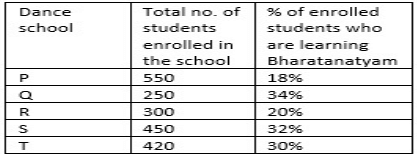Q. What is the ratio of the students who are learning Bharatanatyam from school Q to the total number of students who are not learning Bharatanatyam from school S?

Detailed Solution for SSC CGL Tier-I Mock Test - 43 - Question 72

No. of students who are learning Bharatanatyam from school Q = 250 × 34/100
= 85
No. of students who are not learning Bharatanatyam from school S = 450 × 68/100
= 306
∴ Required ratio = 5 : 18

SSC CGL Tier-I Mock Test - 43 - Question 73

Directions

Study the graph and answer the following questions:Q. In school R, out of the total number of students learning Bharatanatyam, 1/9 are below 15 years of age. If out of the total students who are below 15 years of age 30% are females, then what is the male number of students who below 15 years of age learning Bharatanatyam?

Detailed Solution for SSC CGL Tier-I Mock Test - 43 - Question 73

No. of students who are below 15 years of age in school R = 300 × 20/100
= 60
No. of male students who are learning Bharatanatyam in school R = 60 × 70/100
= 42

SSC CGL Tier-I Mock Test - 43 - Question 74

Directions

Study the graph and answer the following questions:Q. What is the difference between the total number of students learning Bharatanatyam from school P and R to the number of students who are not learning Bharatanatyam from school T?

Detailed Solution for SSC CGL Tier-I Mock Test - 43 - Question 74

P = 550 × 18/100
= 99
R = 300× 20/100 = 60
Total students from P and R
= 99 + 60
= 159
T - 420 × 70/100 = 294
∴ Required difference = 294 - 159 = 135

SSC CGL Tier-I Mock Test - 43 - Question 75

Directions In each question, there are three sentences. Each has a pair of words that are italicised and highlighted. From the italicised and highlighted words, select the most appropriate words (A or B) to form correct sentences. The sentences are followed by options that indicate the words, which may be selected to correctly complete the set of sentences. From the options given, choose the most appropriate one.

The focus on jobs is obviously vital (A)/ peripheral (B). At the root of the crisis is the step (A) / steep (B) fall in the prices of agricultural goods. Growth can come about without any substantial (A) / sustainable (B) job-creation in the formal sectors of the economy, but through improvements in productivity.

Detailed Solution for SSC CGL Tier-I Mock Test - 43 - Question 75

vital means important peripheral means unimportant step means move, pace, stairs steep means sharp, abrupt substantial means significant sustainable means able to be maintained at a certain rate or level

## SSC CGL Tier 1 Mock Test Series

42 docs|103 tests
 Use Code STAYHOME200 and get INR 200 additional OFF Use Coupon Code
Information about SSC CGL Tier-I Mock Test - 43 Page
In this test you can find the Exam questions for SSC CGL Tier-I Mock Test - 43 solved & explained in the simplest way possible. Besides giving Questions and answers for SSC CGL Tier-I Mock Test - 43, EduRev gives you an ample number of Online tests for practice

## SSC CGL Tier 1 Mock Test Series

42 docs|103 tests